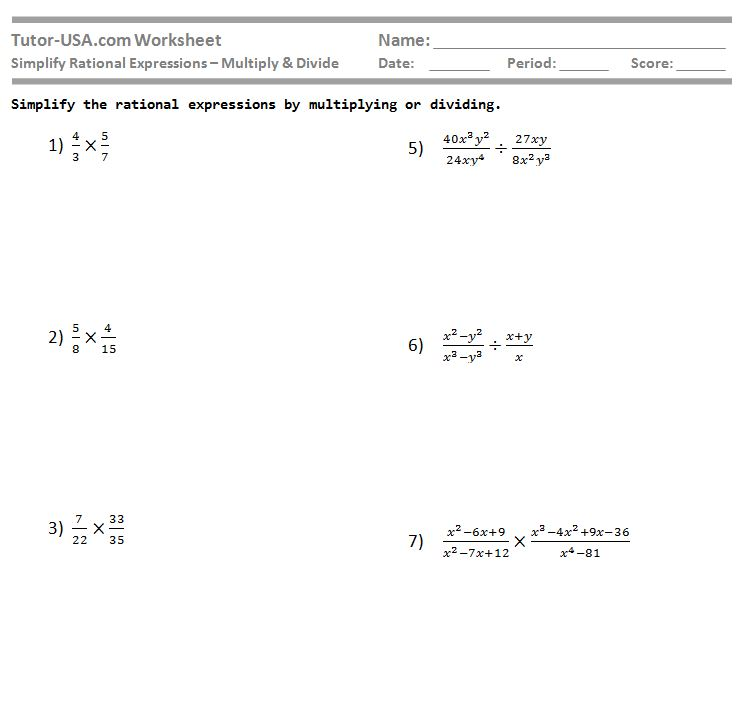# Simplify Rational Expressions - Multiply and Divide Fractions

Worksheet (Algebra)

## Simplify Rational Expressions - Multiply and Divide Fractions Worksheet

In this free algebra worksheet, students must simplify rational expressions. Students are required to multiply and divide algebraic fractions and express the answer in simplest form.

## Lesson:

To multiply and divide fractions. To simplify rational expressions.

12

3

Yes

## Samples:

Tutor-USA.com Worksheet
Simplify Rational Expressions – Multiply & Divide

Simplify the rational expressions by multiplying or dividing

Screenshot: